Next: Naive Bayes text classification Up: Text classification and Naive Previous: Text classification and Naive   Contents   Index

The text classification problem

In text classification, we are given a description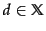of a document, whereis the document space ; and a fixed set of classes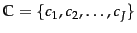. Classes are also called categories or labels . Typically, the document spaceis some type of high-dimensional space, and the classes are human defined for the needs of an application, as in the examples China and documents that talk about multicore computer chips above. We are given a training set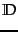of labeled documents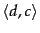, where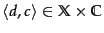. For example: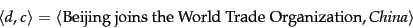(111)

for the one-sentence document Beijing joins the World Trade Organization and the class (or label) China.

Using a learning method or learning algorithm , we then wish to learn a classifier or classification function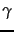that maps documents to classes: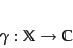(112)

This type of learning is called supervised learning because a supervisor (the human who defines the classes and labels training documents) serves as a teacher directing the learning process. We denote the supervised learning method by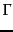and write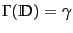. The learning methodtakes the training setas input and returns the learned classification function.

Most names for learning methodsare also used for classifiers. We talk about the Naive Bayes (NB) learning methodwhen we say that Naive Bayes is robust,'' meaning that it can be applied to many different learning problems and is unlikely to produce classifiers that fail catastrophically. But when we say that Naive Bayes had an error rate of 20%,'' we are describing an experiment in which a particular NB classifier(which was produced by the NB learning method) had a 20% error rate in an application.

Figure 13.1 shows an example of text classification from the Reuters-RCV1 collection, introduced in Section 4.2 , page 4.2 . There are six classes (UK, China, ..., sports), each with three training documents. We show a few mnemonic words for each document's content. The training set provides some typical examples for each class, so that we can learn the classification function. Once we have learned, we can apply it to the test set (or test data ), for example, the new document first private Chinese airline whose class is unknown. In Figure 13.1 , the classification function assigns the new document to class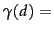China, which is the correct assignment.

The classes in text classification often have some interesting structure such as the hierarchy in Figure 13.1 . There are two instances each of region categories, industry categories, and subject area categories. A hierarchy can be an important aid in solving a classification problem; see Section 15.3.2 for further discussion. Until then, we will make the assumption in the text classification chapters that the classes form a set with no subset relationships between them.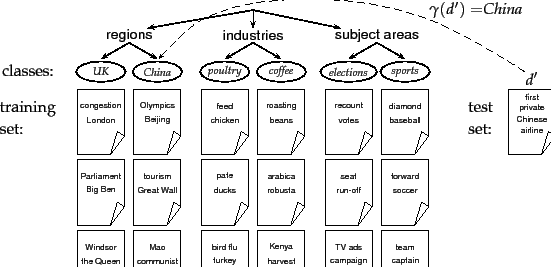Definition eqn:gammadef stipulates that a document is a member of exactly one class. This is not the most appropriate model for the hierarchy in Figure 13.1 . For instance, a document about the 2008 Olympics should be a member of two classes: the China class and the sports class. This type of classification problem is referred to as an any-of problem and we will return to it in Section 14.5 (page). For the time being, we only consider one-of problems where a document is a member of exactly one class.

Our goal in text classification is high accuracy on test data or new data - for example, the newswire articles that we will encounter tomorrow morning in the multicore chip example. It is easy to achieve high accuracy on the training set (e.g., we can simply memorize the labels). But high accuracy on the training set in general does not mean that the classifier will work well on new data in an application. When we use the training set to learn a classifier for test data, we make the assumption that training data and test data are similar or from the same distribution. We defer a precise definition of this notion to Section 14.6 (page).Next: Naive Bayes text classification Up: Text classification and Naive Previous: Text classification and Naive   Contents   Index